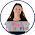# Pythagorean Theorem Proof Without Words

By the time my students reach me, they have already heard of the Pythagorean Theorem.  However, when I introduce right triangles, I always start with a lesson on the Pythagorean Theorem.  I find that many students don’t understand where it comes from and just take it blindly as a formula.
I like to show my students Perigal’s Puzzle.  Basically, it is a proof without words of the Pythagorean Theorem.  First, you start with a right triangle.

Then, you have the areas of each side: a^2, b^2, and c^2.  To make things easy, I will call them Square A, Square B, and Square C.
Cutting apart Square B, you can fit Squares A and B to be the same as Square C.
BAM!  The Pythagorean Theorem works.

Here is a file that you can download for easy cutting :)  Also, if cutting paper isn’t your style, there is a YouTube video you can show.

Enjoy!1.2.